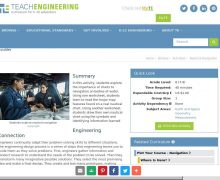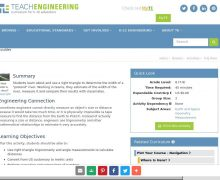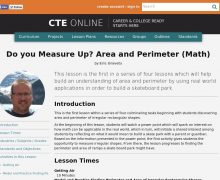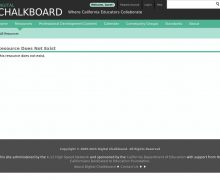• # Math: Measurement And Data

Browse All 22 ResourcesIn this activity, students explore the importance of charts to navigation on bodies of water. Using one worksheet, students learn to read the major map features found on a real nau...In this lesson, students will consider and propose various factors that can be used to make decisions regarding prospective immigrants to the United States of America. Students wil...Juxtaposing the architecture of Ellis island with that of the proposed Trump Wall creates many opportunities for mathematical analysis and frames a discussion of how our nation's a...Students learn about and use a right triangle to determine the width of a "pretend" river. Working in teams, they estimate of the width of the river, measure it and compare their r...This lesson is the first in a series of four lessons which will help build an understanding of area and perimeter by using real world applications in order to build a skateboard pa...Students will see an animated presentation of the proof of the Pythagorean Theorem. This animated PowerPoint presentation uses shearing and the invariance of the area of triangles ...This set of instructional materials and problems helps students understand ratios and proportions, starting with a simple review and progressing into the more advanced territory of...In this session, students will begin to explore the questions "What can be measured?" and "What does it mean to measure something?" Students identify measurable properties of objec...These 20 images give students practice measuring the height and width of various pictures with paperclips. Ready to print, each sheet contains a spot for students to write in their...The classroom poster helps students understand fractions as parts of a whole by illustrating pizzas and fractions of pizzas. These eight images show a whole pizza, a pizza cut in h...In this activity, students explore the importance of charts to navigation on bodies of water. Using one worksheet, students learn to read the major map features found on a real nau...Juxtaposing the architecture of Ellis island with that of the proposed Trump Wall creates many opportunities for mathematical analysis and frames a discussion of how our nation's a...This lesson is the first in a series of four lessons which will help build an understanding of area and perimeter by using real world applications in order to build a skateboard pa...In this lesson, students will consider and propose various factors that can be used to make decisions regarding prospective immigrants to the United States of America. Students wil...Students will see an animated presentation of the proof of the Pythagorean Theorem. This animated PowerPoint presentation uses shearing and the invariance of the area of triangles ...Students learn about and use a right triangle to determine the width of a "pretend" river. Working in teams, they estimate of the width of the river, measure it and compare their r...This set of instructional materials and problems helps students understand ratios and proportions, starting with a simple review and progressing into the more advanced territory of...In this session, students will begin to explore the questions "What can be measured?" and "What does it mean to measure something?" Students identify measurable properties of objec...These 20 images give students practice measuring the height and width of various pictures with paperclips. Ready to print, each sheet contains a spot for students to write in their...